Next:6.2.2 Binomial Amortized AnalysisUp:6.2 Binomial QueuesPrevious:6.2 Binomial Queues

## 6.2.1 Binomial Queue Operations

Find-min

This is implemented by scanning the roots of all the trees. Since there are at most log n different trees, this leads to a worstcase complexity of 0(log n).

Alternatively, one can keep track of the current minimum and perform find-min in 0(1) time if we remember to update the minimum if it changes during other operations.

Merge

Let H1: 6 elements

H2: 7 elements

We are now left with

1 tree of height 0

3 trees of height 2

Note that of the 3 binomial trees of height 2, we could have any pair to get another binomial heap. Since merging two binomial trees takes constant time and there are 0(log n) binomial trees, merge takes 0(log n) in the worstcase. See Figures 6.7 and 6.8 for two examples.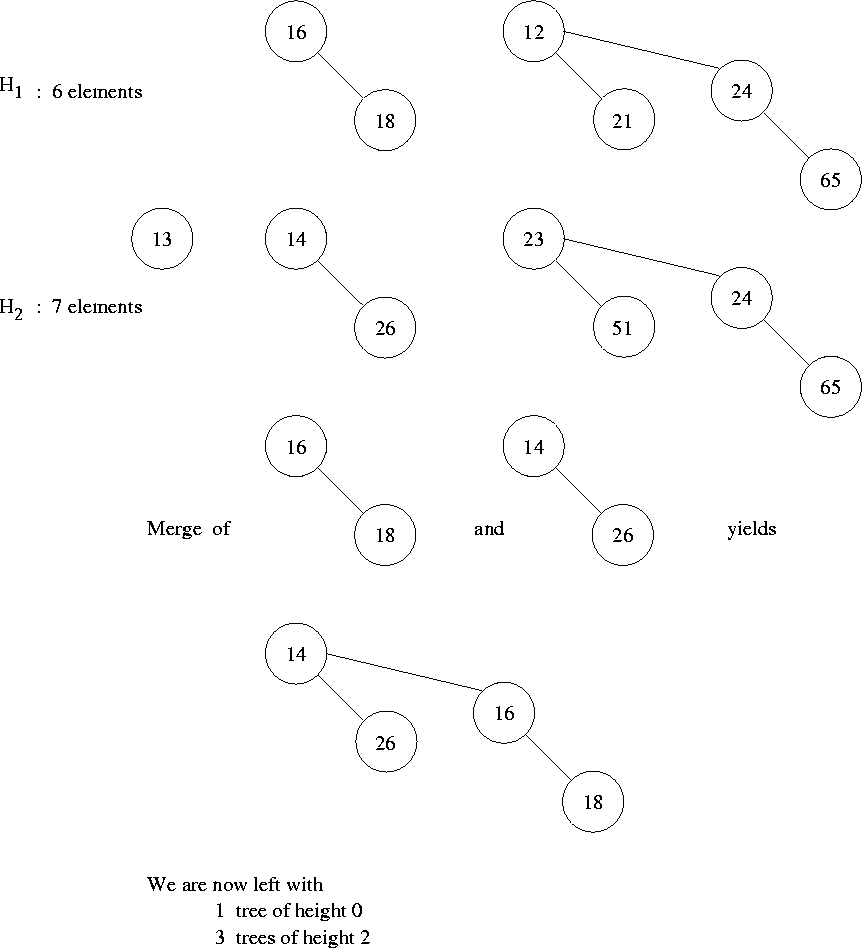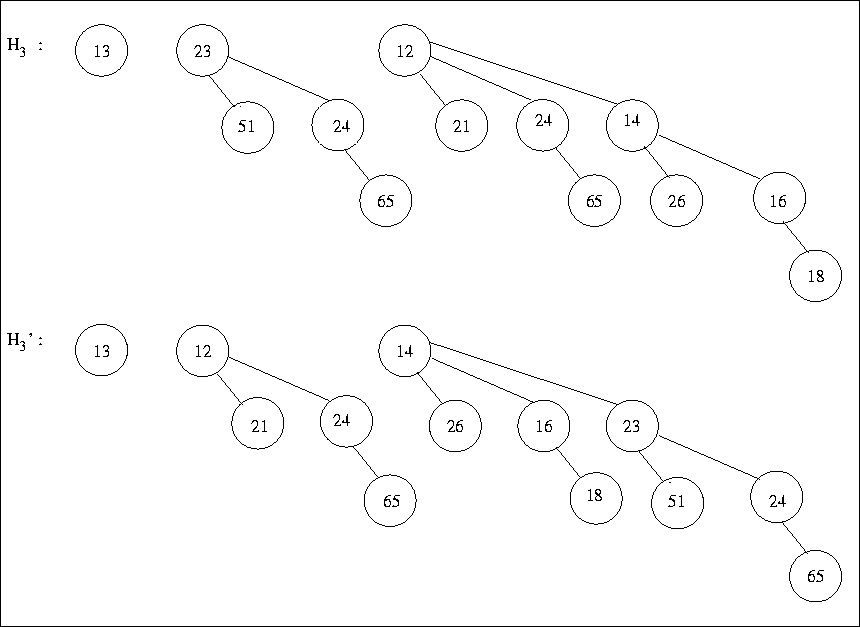Insertion

This is a special case of merging since we merely create a one-node tree and perform a merge.

Worstcase complexity therefore will be O(log n).

More precisely; if the priority queue into which the element is being inserted has the property that the smallest nonexistent binomial tree is Bi, the running time is proportional to i + 1.

Eg: Insertion into H3 (which has 13 elements) terminates in two steps.

Since each tree of a particular degree in a binomial queue is present with probability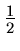, if we define the random variable X as representing the number of steps in an insert operation, then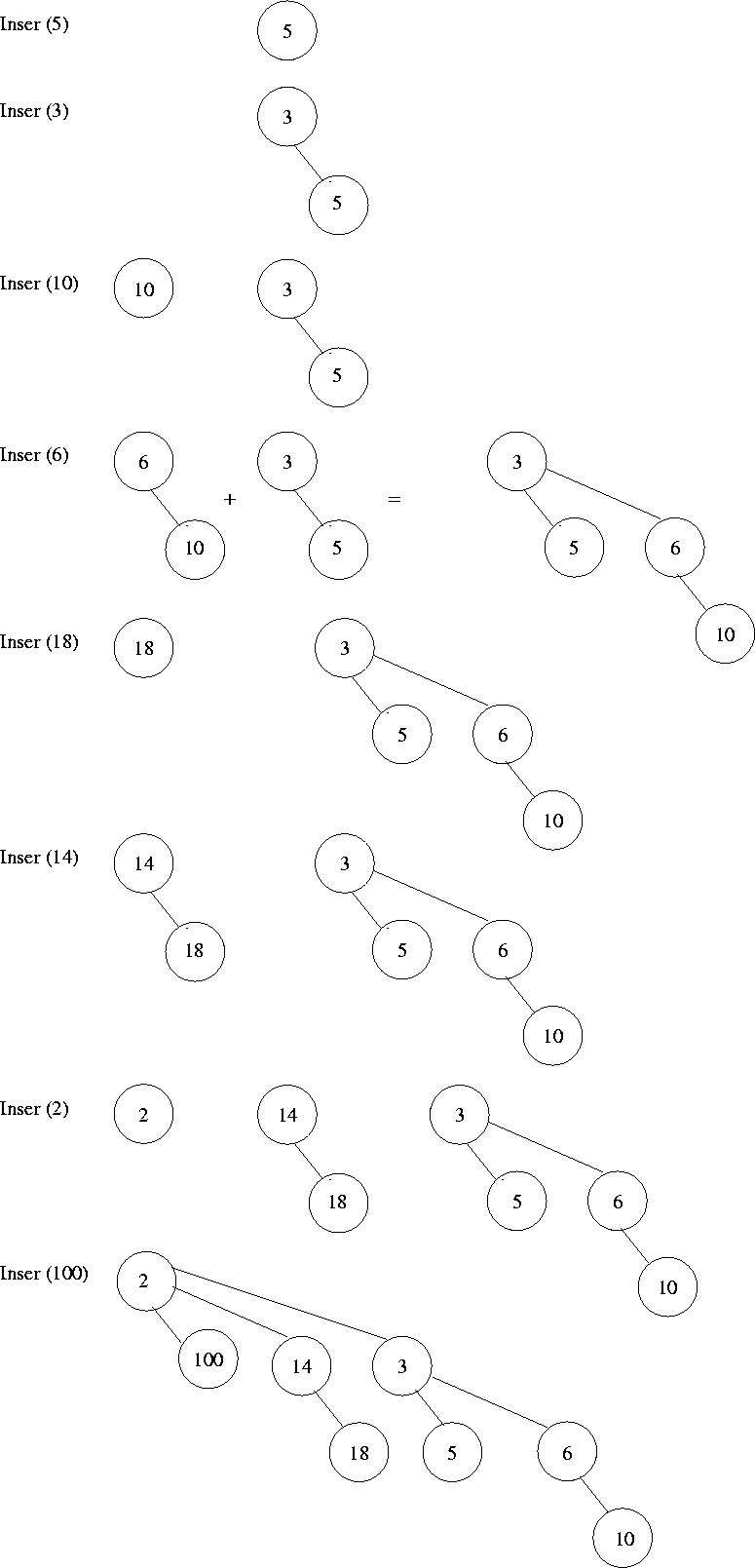X = 1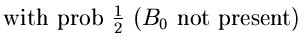= 2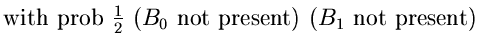= 3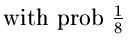*15pt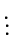Thus average number of steps in an insert operation = 2

Thus we expect an insertion to terminate in two steps on the average. Further more, performing n inserts on an initially empty binomial queue will take 0(n) worstcase time.

See Figures 6.9 and 6.10 for an example.

Deletemin

• First find the binomial tree with the smallest root. Let this be Bk. Let H be the original priority queue.
• Remove Bk from the forest in H forming another binomial queue H'.
• Now remove the root of Bk creating binomial trees B0, B1, ..., Bk - 1, which collectively form a binomial queue H''.
• Now, merge H' and H''.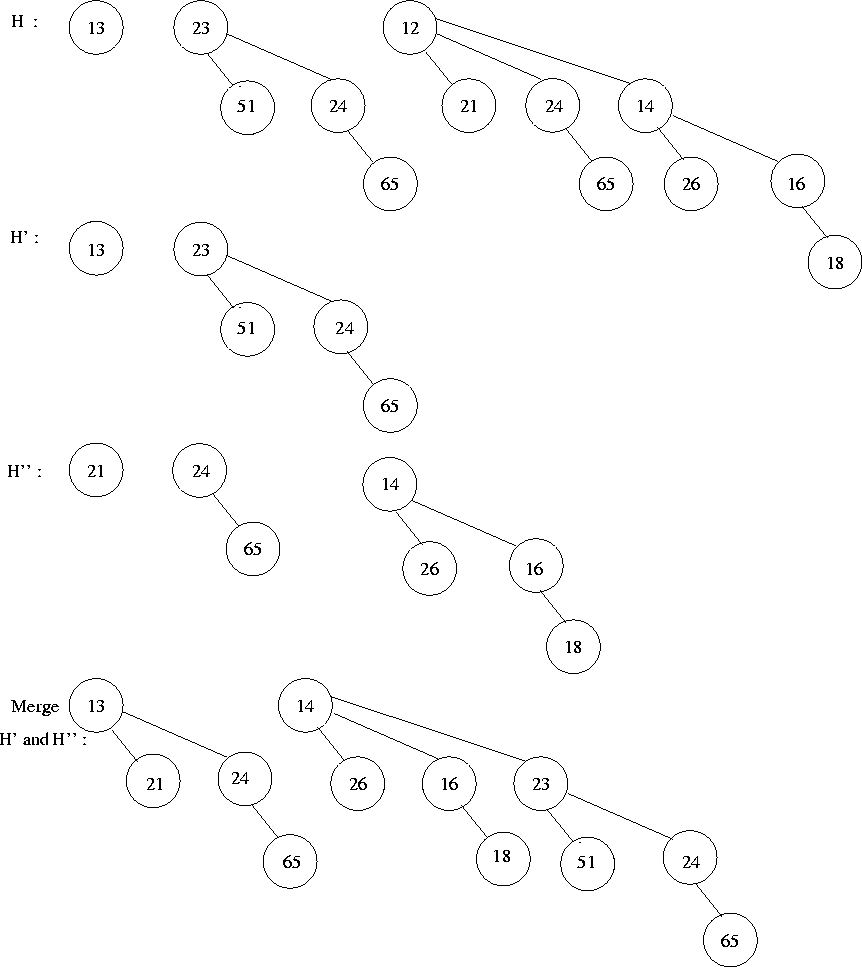It is easy to see that the worstcase complexity of deletemin is 0(log n).

Implementation of a Binomial Queue

• deletemin operation requires ability to find all subtrees of the root. Thus children of each node should be available (say a linked list)
• deletemin requires that the children be ordered by the size of their subtrees.
• we need to make sure it is easy to merge tress. Two binomial trees can be merged only if they have the same size, hence the size of the tree must be stored in the root. While merging, one of the trees becomes the last child of the other, so we should keep track of the last child of each node. A good data structure to use is a circular doubly linked list what each node is of the following form:
•
 data first left right rank No. of child sibling sibling childrenNext:6.2.2 Binomial Amortized AnalysisUp:6.2 Binomial QueuesPrevious:6.2 Binomial Queues
eEL,CSA_Dept,IISc,Bangalore